# Shapes¶

In this section we look at different shapes. Shapes are attached to bodies. There are three basic shape classes:

• Circle
• Segment
• Poly

## The Box class¶

Many of the simulations require a static box to contain the dynamic elements. So let’s define the `Box` class which takes 2 diagonal end points to define a box:

```class Box:
def __init__(self, p0=(10, 10), p1=(690, 230), d=2):
x0, y0 = p0
x1, y1 = p1
pts = [(x0, y0), (x1, y0), (x1, y1), (x0, y1)]
for i in range(4):
segment = pymunk.Segment(space.static_body, pts[i], pts[(i+1)%4], d)
segment.elasticity = 1
segment.friction = 1
```

The new class library for this section can be found here:

`shape.py`

## A simple compound shape¶

Two separate shapes can be attached to a body. To distinguish them we give them different colors.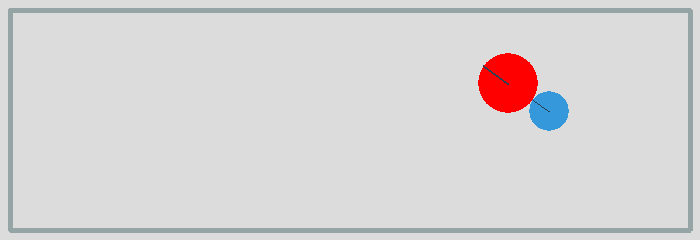`shape1.py`

## A moving segment¶

Segments are linear elements which have a radius. The following code represents a segment of length 100 with its center of gravity at the center:

```body = pymunk.Body(mass=1, moment=1000)
body.position = (100, 200)
body.apply_impulse_at_local_point((100, 0), (0, 1))

shape = pymunk.Segment(body, (-50, 0), (50, 0), radius=10)
shape.elasticity = 0.999
```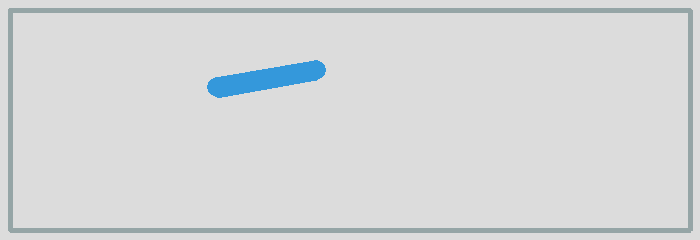`shape2.py`

## L-shaped segment¶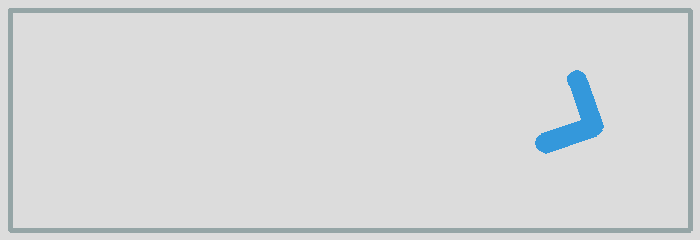`shape3.py`

## Multiple L-shaped segment¶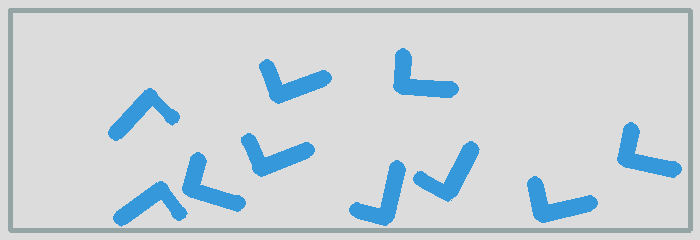`shape4.py`

## Triangles¶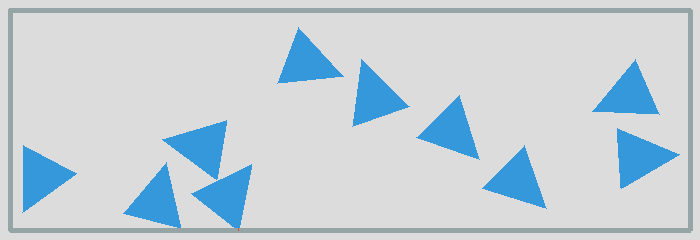`shape6.py`

## Conveyer belt¶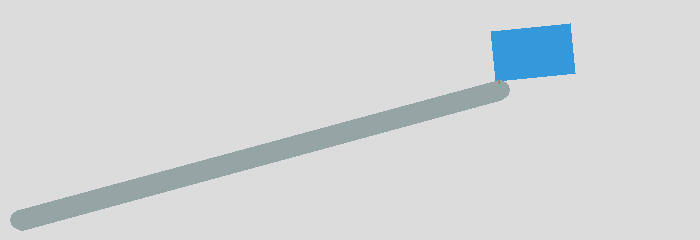`shape7.py`

## Pivot joint¶`shape8.py`

## Damped spring¶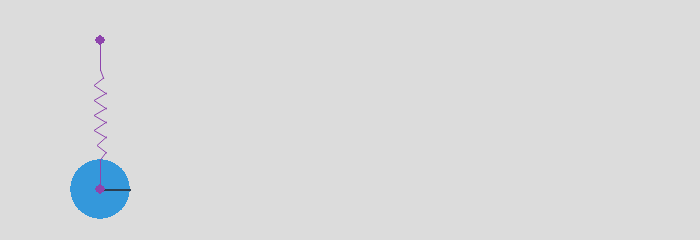`shape9.py`